# Fundamental Theorems of Asset Pricing

15 Nov 2019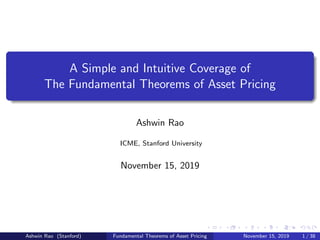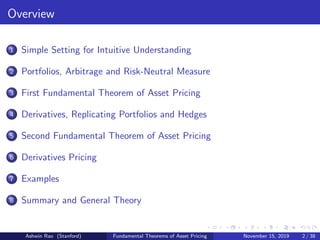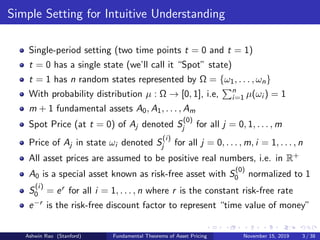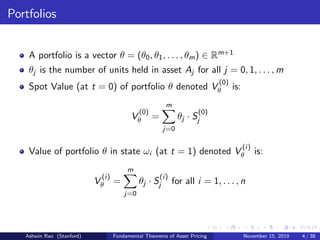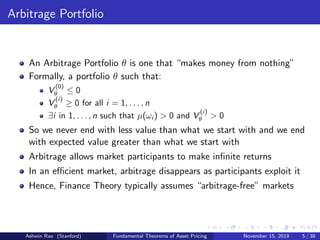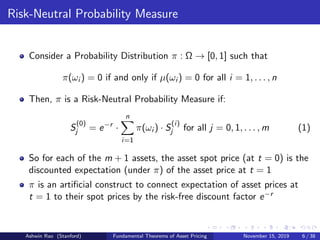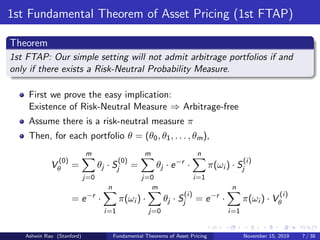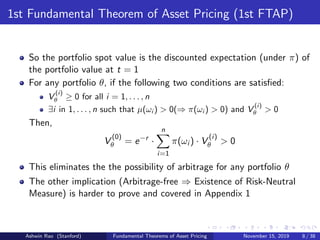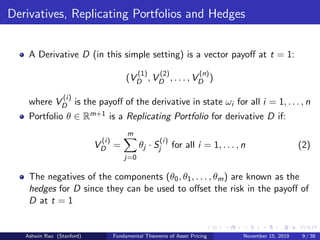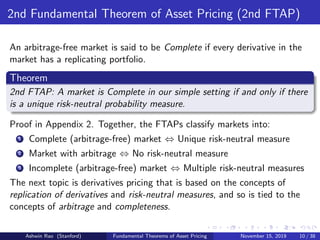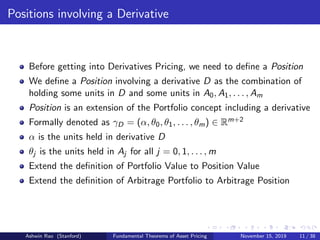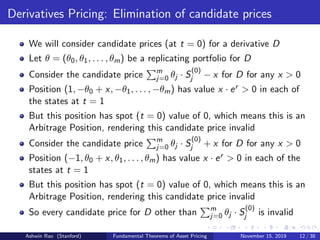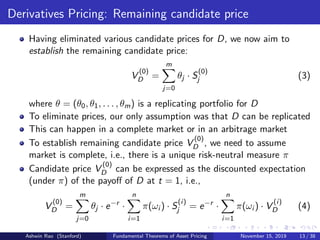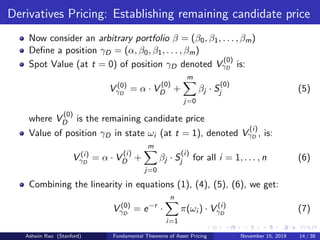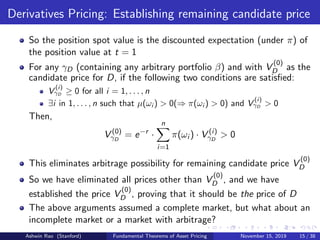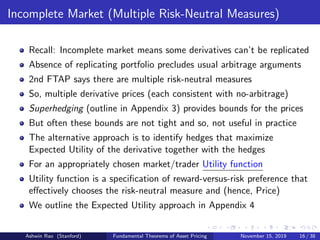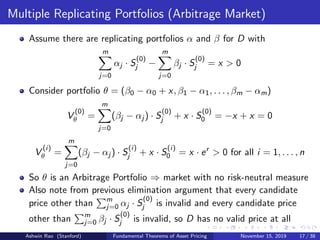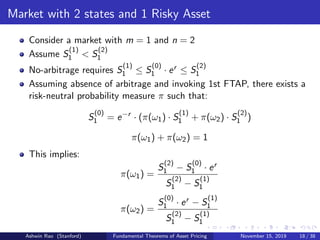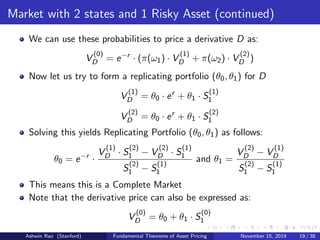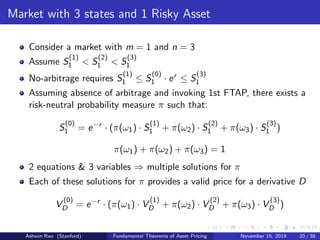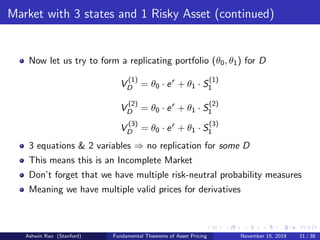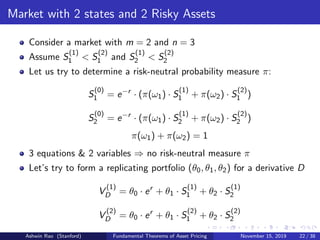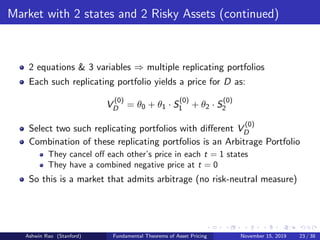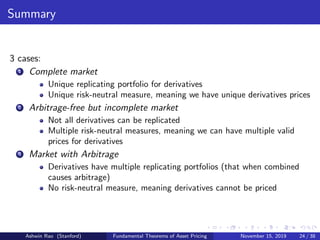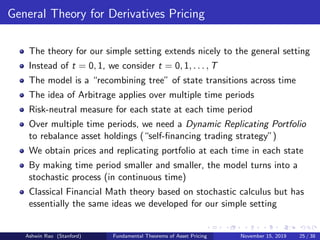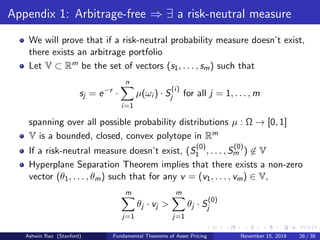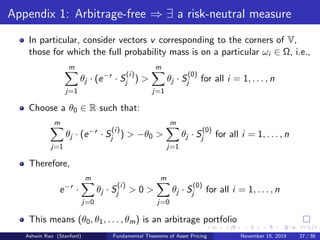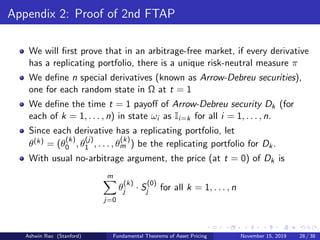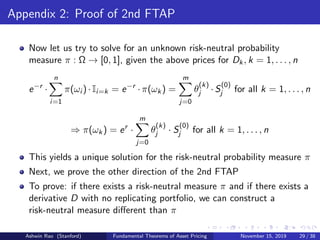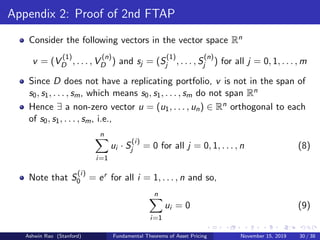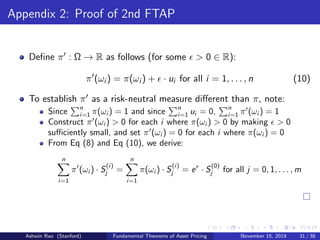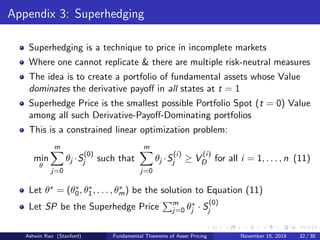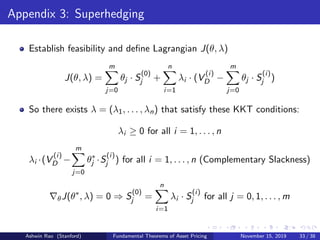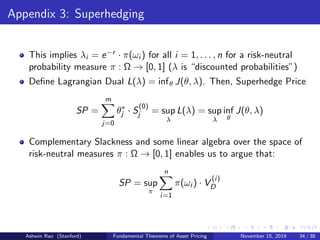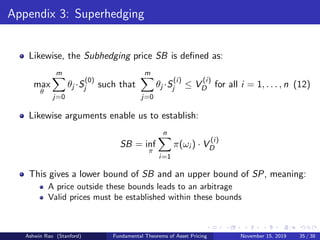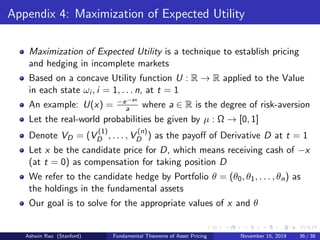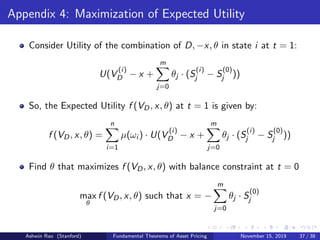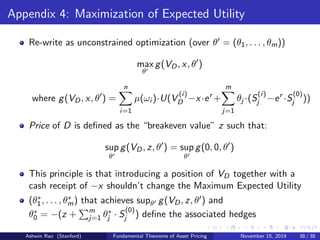1 sur 38

### Fundamental Theorems of Asset Pricing

• 1. A Simple and Intuitive Coverage of The Fundamental Theorems of Asset Pricing Ashwin Rao ICME, Stanford University November 15, 2019 Ashwin Rao (Stanford) Fundamental Theorems of Asset Pricing November 15, 2019 1 / 38
• 2. Overview 1 Simple Setting for Intuitive Understanding 2 Portfolios, Arbitrage and Risk-Neutral Measure 3 First Fundamental Theorem of Asset Pricing 4 Derivatives, Replicating Portfolios and Hedges 5 Second Fundamental Theorem of Asset Pricing 6 Derivatives Pricing 7 Examples 8 Summary and General Theory Ashwin Rao (Stanford) Fundamental Theorems of Asset Pricing November 15, 2019 2 / 38
• 3. Simple Setting for Intuitive Understanding Single-period setting (two time points t = 0 and t = 1) t = 0 has a single state (we’ll call it “Spot” state) t = 1 has n random states represented by Ω = {ω1, . . . , ωn} With probability distribution µ : Ω → [0, 1], i.e, n i=1 µ(ωi ) = 1 m + 1 fundamental assets A0, A1, . . . , Am Spot Price (at t = 0) of Aj denoted S (0) j for all j = 0, 1, . . . , m Price of Aj in state ωi denoted S (i) j for all j = 0, . . . , m, i = 1, . . . , n All asset prices are assumed to be positive real numbers, i.e. in R+ A0 is a special asset known as risk-free asset with S (0) 0 normalized to 1 S (i) 0 = er for all i = 1, . . . , n where r is the constant risk-free rate e−r is the risk-free discount factor to represent “time value of money” Ashwin Rao (Stanford) Fundamental Theorems of Asset Pricing November 15, 2019 3 / 38
• 4. Portfolios A portfolio is a vector θ = (θ0, θ1, . . . , θm) ∈ Rm+1 θj is the number of units held in asset Aj for all j = 0, 1, . . . , m Spot Value (at t = 0) of portfolio θ denoted V (0) θ is: V (0) θ = m j=0 θj · S (0) j Value of portfolio θ in state ωi (at t = 1) denoted V (i) θ is: V (i) θ = m j=0 θj · S (i) j for all i = 1, . . . , n Ashwin Rao (Stanford) Fundamental Theorems of Asset Pricing November 15, 2019 4 / 38
• 5. Arbitrage Portfolio An Arbitrage Portfolio θ is one that “makes money from nothing” Formally, a portfolio θ such that: V (0) θ ≤ 0 V (i) θ ≥ 0 for all i = 1, . . . , n ∃i in 1, . . . , n such that µ(ωi ) > 0 and V (i) θ > 0 So we never end with less value than what we start with and we end with expected value greater than what we start with Arbitrage allows market participants to make inﬁnite returns In an eﬃcient market, arbitrage disappears as participants exploit it Hence, Finance Theory typically assumes “arbitrage-free” markets Ashwin Rao (Stanford) Fundamental Theorems of Asset Pricing November 15, 2019 5 / 38
• 6. Risk-Neutral Probability Measure Consider a Probability Distribution π : Ω → [0, 1] such that π(ωi ) = 0 if and only if µ(ωi ) = 0 for all i = 1, . . . , n Then, π is a Risk-Neutral Probability Measure if: S (0) j = e−r · n i=1 π(ωi ) · S (i) j for all j = 0, 1, . . . , m (1) So for each of the m + 1 assets, the asset spot price (at t = 0) is the discounted expectation (under π) of the asset price at t = 1 π is an artiﬁcial construct to connect expectation of asset prices at t = 1 to their spot prices by the risk-free discount factor e−r Ashwin Rao (Stanford) Fundamental Theorems of Asset Pricing November 15, 2019 6 / 38
• 7. 1st Fundamental Theorem of Asset Pricing (1st FTAP) Theorem 1st FTAP: Our simple setting will not admit arbitrage portfolios if and only if there exists a Risk-Neutral Probability Measure. First we prove the easy implication: Existence of Risk-Neutral Measure ⇒ Arbitrage-free Assume there is a risk-neutral measure π Then, for each portfolio θ = (θ0, θ1, . . . , θm), V (0) θ = m j=0 θj · S (0) j = m j=0 θj · e−r · n i=1 π(ωi ) · S (i) j = e−r · n i=1 π(ωi ) · m j=0 θj · S (i) j = e−r · n i=1 π(ωi ) · V (i) θ Ashwin Rao (Stanford) Fundamental Theorems of Asset Pricing November 15, 2019 7 / 38
• 8. 1st Fundamental Theorem of Asset Pricing (1st FTAP) So the portfolio spot value is the discounted expectation (under π) of the portfolio value at t = 1 For any portfolio θ, if the following two conditions are satisﬁed: V (i) θ ≥ 0 for all i = 1, . . . , n ∃i in 1, . . . , n such that µ(ωi ) > 0(⇒ π(ωi ) > 0) and V (i) θ > 0 Then, V (0) θ = e−r · n i=1 π(ωi ) · V (i) θ > 0 This eliminates the the possibility of arbitrage for any portfolio θ The other implication (Arbitrage-free ⇒ Existence of Risk-Neutral Measure) is harder to prove and covered in Appendix 1 Ashwin Rao (Stanford) Fundamental Theorems of Asset Pricing November 15, 2019 8 / 38
• 9. Derivatives, Replicating Portfolios and Hedges A Derivative D (in this simple setting) is a vector payoﬀ at t = 1: (V (1) D , V (2) D , . . . , V (n) D ) where V (i) D is the payoﬀ of the derivative in state ωi for all i = 1, . . . , n Portfolio θ ∈ Rm+1 is a Replicating Portfolio for derivative D if: V (i) D = m j=0 θj · S (i) j for all i = 1, . . . , n (2) The negatives of the components (θ0, θ1, . . . , θm) are known as the hedges for D since they can be used to oﬀset the risk in the payoﬀ of D at t = 1 Ashwin Rao (Stanford) Fundamental Theorems of Asset Pricing November 15, 2019 9 / 38
• 10. 2nd Fundamental Theorem of Asset Pricing (2nd FTAP) An arbitrage-free market is said to be Complete if every derivative in the market has a replicating portfolio. Theorem 2nd FTAP: A market is Complete in our simple setting if and only if there is a unique risk-neutral probability measure. Proof in Appendix 2. Together, the FTAPs classify markets into: 1 Complete (arbitrage-free) market ⇔ Unique risk-neutral measure 2 Market with arbitrage ⇔ No risk-neutral measure 3 Incomplete (arbitrage-free) market ⇔ Multiple risk-neutral measures The next topic is derivatives pricing that is based on the concepts of replication of derivatives and risk-neutral measures, and so is tied to the concepts of arbitrage and completeness. Ashwin Rao (Stanford) Fundamental Theorems of Asset Pricing November 15, 2019 10 / 38
• 11. Positions involving a Derivative Before getting into Derivatives Pricing, we need to deﬁne a Position We deﬁne a Position involving a derivative D as the combination of holding some units in D and some units in A0, A1, . . . , Am Position is an extension of the Portfolio concept including a derivative Formally denoted as γD = (α, θ0, θ1, . . . , θm) ∈ Rm+2 α is the units held in derivative D θj is the units held in Aj for all j = 0, 1, . . . , m Extend the deﬁnition of Portfolio Value to Position Value Extend the deﬁnition of Arbitrage Portfolio to Arbitrage Position Ashwin Rao (Stanford) Fundamental Theorems of Asset Pricing November 15, 2019 11 / 38
• 12. Derivatives Pricing: Elimination of candidate prices We will consider candidate prices (at t = 0) for a derivative D Let θ = (θ0, θ1, . . . , θm) be a replicating portfolio for D Consider the candidate price m j=0 θj · S (0) j − x for D for any x > 0 Position (1, −θ0 + x, −θ1, . . . , −θm) has value x · er > 0 in each of the states at t = 1 But this position has spot (t = 0) value of 0, which means this is an Arbitrage Position, rendering this candidate price invalid Consider the candidate price m j=0 θj · S (0) j + x for D for any x > 0 Position (−1, θ0 + x, θ1, . . . , θm) has value x · er > 0 in each of the states at t = 1 But this position has spot (t = 0) value of 0, which means this is an Arbitrage Position, rendering this candidate price invalid So every candidate price for D other than m j=0 θj · S (0) j is invalid Ashwin Rao (Stanford) Fundamental Theorems of Asset Pricing November 15, 2019 12 / 38
• 13. Derivatives Pricing: Remaining candidate price Having eliminated various candidate prices for D, we now aim to establish the remaining candidate price: V (0) D = m j=0 θj · S (0) j (3) where θ = (θ0, θ1, . . . , θm) is a replicating portfolio for D To eliminate prices, our only assumption was that D can be replicated This can happen in a complete market or in an arbitrage market To establish remaining candidate price V (0) D , we need to assume market is complete, i.e., there is a unique risk-neutral measure π Candidate price V (0) D can be expressed as the discounted expectation (under π) of the payoﬀ of D at t = 1, i.e., V (0) D = m j=0 θj · e−r · n i=1 π(ωi ) · S (i) j = e−r · n i=1 π(ωi ) · V (i) D (4) Ashwin Rao (Stanford) Fundamental Theorems of Asset Pricing November 15, 2019 13 / 38
• 14. Derivatives Pricing: Establishing remaining candidate price Now consider an arbitrary portfolio β = (β0, β1, . . . , βm) Deﬁne a position γD = (α, β0, β1, . . . , βm) Spot Value (at t = 0) of position γD denoted V (0) γD is: V (0) γD = α · V (0) D + m j=0 βj · S (0) j (5) where V (0) D is the remaining candidate price Value of position γD in state ωi (at t = 1), denoted V (i) γD , is: V (i) γD = α · V (i) D + m j=0 βj · S (i) j for all i = 1, . . . , n (6) Combining the linearity in equations (1), (4), (5), (6), we get: V (0) γD = e−r · n i=1 π(ωi ) · V (i) γD (7) Ashwin Rao (Stanford) Fundamental Theorems of Asset Pricing November 15, 2019 14 / 38
• 15. Derivatives Pricing: Establishing remaining candidate price So the position spot value is the discounted expectation (under π) of the position value at t = 1 For any γD (containing any arbitrary portfolio β) and with V (0) D as the candidate price for D, if the following two conditions are satisﬁed: V (i) γD ≥ 0 for all i = 1, . . . , n ∃i in 1, . . . , n such that µ(ωi ) > 0(⇒ π(ωi ) > 0) and V (i) γD > 0 Then, V (0) γD = e−r · n i=1 π(ωi ) · V (i) γD > 0 This eliminates arbitrage possibility for remaining candidate price V (0) D So we have eliminated all prices other than V (0) D , and we have established the price V (0) D , proving that it should be the price of D The above arguments assumed a complete market, but what about an incomplete market or a market with arbitrage? Ashwin Rao (Stanford) Fundamental Theorems of Asset Pricing November 15, 2019 15 / 38
• 16. Incomplete Market (Multiple Risk-Neutral Measures) Recall: Incomplete market means some derivatives can’t be replicated Absence of replicating portfolio precludes usual arbitrage arguments 2nd FTAP says there are multiple risk-neutral measures So, multiple derivative prices (each consistent with no-arbitrage) Superhedging (outline in Appendix 3) provides bounds for the prices But often these bounds are not tight and so, not useful in practice The alternative approach is to identify hedges that maximize Expected Utility of the derivative together with the hedges For an appropriately chosen market/trader Utility function Utility function is a speciﬁcation of reward-versus-risk preference that eﬀectively chooses the risk-neutral measure and (hence, Price) We outline the Expected Utility approach in Appendix 4 Ashwin Rao (Stanford) Fundamental Theorems of Asset Pricing November 15, 2019 16 / 38
• 17. Multiple Replicating Portfolios (Arbitrage Market) Assume there are replicating portfolios α and β for D with m j=0 αj · S (0) j − m j=0 βj · S (0) j = x > 0 Consider portfolio θ = (β0 − α0 + x, β1 − α1, . . . , βm − αm) V (0) θ = m j=0 (βj − αj ) · S (0) j + x · S (0) 0 = −x + x = 0 V (i) θ = m j=0 (βj − αj ) · S (i) j + x · S (i) 0 = x · er > 0 for all i = 1, . . . , n So θ is an Arbitrage Portfolio ⇒ market with no risk-neutral measure Also note from previous elimination argument that every candidate price other than m j=0 αj · S (0) j is invalid and every candidate price other than m j=0 βj · S (0) j is invalid, so D has no valid price at all Ashwin Rao (Stanford) Fundamental Theorems of Asset Pricing November 15, 2019 17 / 38
• 18. Market with 2 states and 1 Risky Asset Consider a market with m = 1 and n = 2 Assume S (1) 1 < S (2) 1 No-arbitrage requires S (1) 1 ≤ S (0) 1 · er ≤ S (2) 1 Assuming absence of arbitrage and invoking 1st FTAP, there exists a risk-neutral probability measure π such that: S (0) 1 = e−r · (π(ω1) · S (1) 1 + π(ω2) · S (2) 1 ) π(ω1) + π(ω2) = 1 This implies: π(ω1) = S (2) 1 − S (0) 1 · er S (2) 1 − S (1) 1 π(ω2) = S (0) 1 · er − S (1) 1 S (2) 1 − S (1) 1 Ashwin Rao (Stanford) Fundamental Theorems of Asset Pricing November 15, 2019 18 / 38
• 19. Market with 2 states and 1 Risky Asset (continued) We can use these probabilities to price a derivative D as: V (0) D = e−r · (π(ω1) · V (1) D + π(ω2) · V (2) D ) Now let us try to form a replicating portfolio (θ0, θ1) for D V (1) D = θ0 · er + θ1 · S (1) 1 V (2) D = θ0 · er + θ1 · S (2) 1 Solving this yields Replicating Portfolio (θ0, θ1) as follows: θ0 = e−r · V (1) D · S (2) 1 − V (2) D · S (1) 1 S (2) 1 − S (1) 1 and θ1 = V (2) D − V (1) D S (2) 1 − S (1) 1 This means this is a Complete Market Note that the derivative price can also be expressed as: V (0) D = θ0 + θ1 · S (0) 1 Ashwin Rao (Stanford) Fundamental Theorems of Asset Pricing November 15, 2019 19 / 38
• 20. Market with 3 states and 1 Risky Asset Consider a market with m = 1 and n = 3 Assume S (1) 1 < S (2) 1 < S (3) 1 No-arbitrage requires S (1) 1 ≤ S (0) 1 · er ≤ S (3) 1 Assuming absence of arbitrage and invoking 1st FTAP, there exists a risk-neutral probability measure π such that: S (0) 1 = e−r · (π(ω1) · S (1) 1 + π(ω2) · S (2) 1 + π(ω3) · S (3) 1 ) π(ω1) + π(ω2) + π(ω3) = 1 2 equations & 3 variables ⇒ multiple solutions for π Each of these solutions for π provides a valid price for a derivative D V (0) D = e−r · (π(ω1) · V (1) D + π(ω2) · V (2) D + π(ω3) · V (3) D ) Ashwin Rao (Stanford) Fundamental Theorems of Asset Pricing November 15, 2019 20 / 38
• 21. Market with 3 states and 1 Risky Asset (continued) Now let us try to form a replicating portfolio (θ0, θ1) for D V (1) D = θ0 · er + θ1 · S (1) 1 V (2) D = θ0 · er + θ1 · S (2) 1 V (3) D = θ0 · er + θ1 · S (3) 1 3 equations & 2 variables ⇒ no replication for some D This means this is an Incomplete Market Don’t forget that we have multiple risk-neutral probability measures Meaning we have multiple valid prices for derivatives Ashwin Rao (Stanford) Fundamental Theorems of Asset Pricing November 15, 2019 21 / 38
• 22. Market with 2 states and 2 Risky Assets Consider a market with m = 2 and n = 3 Assume S (1) 1 < S (2) 1 and S (1) 2 < S (2) 2 Let us try to determine a risk-neutral probability measure π: S (0) 1 = e−r · (π(ω1) · S (1) 1 + π(ω2) · S (2) 1 ) S (0) 2 = e−r · (π(ω1) · S (1) 2 + π(ω2) · S (2) 2 ) π(ω1) + π(ω2) = 1 3 equations & 2 variables ⇒ no risk-neutral measure π Let’s try to form a replicating portfolio (θ0, θ1, θ2) for a derivative D V (1) D = θ0 · er + θ1 · S (1) 1 + θ2 · S (1) 2 V (2) D = θ0 · er + θ1 · S (2) 1 + θ2 · S (2) 2 Ashwin Rao (Stanford) Fundamental Theorems of Asset Pricing November 15, 2019 22 / 38
• 23. Market with 2 states and 2 Risky Assets (continued) 2 equations & 3 variables ⇒ multiple replicating portfolios Each such replicating portfolio yields a price for D as: V (0) D = θ0 + θ1 · S (0) 1 + θ2 · S (0) 2 Select two such replicating portfolios with diﬀerent V (0) D Combination of these replicating portfolios is an Arbitrage Portfolio They cancel oﬀ each other’s price in each t = 1 states They have a combined negative price at t = 0 So this is a market that admits arbitrage (no risk-neutral measure) Ashwin Rao (Stanford) Fundamental Theorems of Asset Pricing November 15, 2019 23 / 38
• 24. Summary 3 cases: 1 Complete market Unique replicating portfolio for derivatives Unique risk-neutral measure, meaning we have unique derivatives prices 2 Arbitrage-free but incomplete market Not all derivatives can be replicated Multiple risk-neutral measures, meaning we can have multiple valid prices for derivatives 3 Market with Arbitrage Derivatives have multiple replicating portfolios (that when combined causes arbitrage) No risk-neutral measure, meaning derivatives cannot be priced Ashwin Rao (Stanford) Fundamental Theorems of Asset Pricing November 15, 2019 24 / 38
• 25. General Theory for Derivatives Pricing The theory for our simple setting extends nicely to the general setting Instead of t = 0, 1, we consider t = 0, 1, . . . , T The model is a “recombining tree” of state transitions across time The idea of Arbitrage applies over multiple time periods Risk-neutral measure for each state at each time period Over multiple time periods, we need a Dynamic Replicating Portfolio to rebalance asset holdings (“self-ﬁnancing trading strategy”) We obtain prices and replicating portfolio at each time in each state By making time period smaller and smaller, the model turns into a stochastic process (in continuous time) Classical Financial Math theory based on stochastic calculus but has essentially the same ideas we developed for our simple setting Ashwin Rao (Stanford) Fundamental Theorems of Asset Pricing November 15, 2019 25 / 38
• 26. Appendix 1: Arbitrage-free ⇒ ∃ a risk-neutral measure We will prove that if a risk-neutral probability measure doesn’t exist, there exists an arbitrage portfolio Let V ⊂ Rm be the set of vectors (s1, . . . , sm) such that sj = e−r · n i=1 µ(ωi ) · S (i) j for all j = 1, . . . , m spanning over all possible probability distributions µ : Ω → [0, 1] V is a bounded, closed, convex polytope in Rm If a risk-neutral measure doesn’t exist, (S (0) 1 , . . . , S (0) m ) ∈ V Hyperplane Separation Theorem implies that there exists a non-zero vector (θ1, . . . , θm) such that for any v = (v1, . . . , vm) ∈ V, m j=1 θj · vj > m j=1 θj · S (0) j Ashwin Rao (Stanford) Fundamental Theorems of Asset Pricing November 15, 2019 26 / 38
• 27. Appendix 1: Arbitrage-free ⇒ ∃ a risk-neutral measure In particular, consider vectors v corresponding to the corners of V, those for which the full probability mass is on a particular ωi ∈ Ω, i.e., m j=1 θj · (e−r · S (i) j ) > m j=1 θj · S (0) j for all i = 1, . . . , n Choose a θ0 ∈ R such that: m j=1 θj · (e−r · S (i) j ) > −θ0 > m j=1 θj · S (0) j for all i = 1, . . . , n Therefore, e−r · m j=0 θj · S (i) j > 0 > m j=0 θj · S (0) j for all i = 1, . . . , n This means (θ0, θ1, . . . , θm) is an arbitrage portfolio Ashwin Rao (Stanford) Fundamental Theorems of Asset Pricing November 15, 2019 27 / 38
• 28. Appendix 2: Proof of 2nd FTAP We will ﬁrst prove that in an arbitrage-free market, if every derivative has a replicating portfolio, there is a unique risk-neutral measure π We deﬁne n special derivatives (known as Arrow-Debreu securities), one for each random state in Ω at t = 1 We deﬁne the time t = 1 payoﬀ of Arrow-Debreu security Dk (for each of k = 1, . . . , n) in state ωi as Ii=k for all i = 1, . . . , n. Since each derivative has a replicating portfolio, let θ(k) = (θ (k) 0 , θ (j) 1 , . . . , θ (k) m ) be the replicating portfolio for Dk. With usual no-arbitrage argument, the price (at t = 0) of Dk is m j=0 θ (k) j · S (0) j for all k = 1, . . . , n Ashwin Rao (Stanford) Fundamental Theorems of Asset Pricing November 15, 2019 28 / 38
• 29. Appendix 2: Proof of 2nd FTAP Now let us try to solve for an unknown risk-neutral probability measure π : Ω → [0, 1], given the above prices for Dk, k = 1, . . . , n e−r · n i=1 π(ωi )·Ii=k = e−r ·π(ωk) = m j=0 θ (k) j ·S (0) j for all k = 1, . . . , n ⇒ π(ωk) = er · m j=0 θ (k) j · S (0) j for all k = 1, . . . , n This yields a unique solution for the risk-neutral probability measure π Next, we prove the other direction of the 2nd FTAP To prove: if there exists a risk-neutral measure π and if there exists a derivative D with no replicating portfolio, we can construct a risk-neutral measure diﬀerent than π Ashwin Rao (Stanford) Fundamental Theorems of Asset Pricing November 15, 2019 29 / 38
• 30. Appendix 2: Proof of 2nd FTAP Consider the following vectors in the vector space Rn v = (V (1) D , . . . , V (n) D ) and sj = (S (1) j , . . . , S (n) j ) for all j = 0, 1, . . . , m Since D does not have a replicating portfolio, v is not in the span of s0, s1, . . . , sm, which means s0, s1, . . . , sm do not span Rn Hence ∃ a non-zero vector u = (u1, . . . , un) ∈ Rn orthogonal to each of s0, s1, . . . , sm, i.e., n i=1 ui · S (i) j = 0 for all j = 0, 1, . . . , n (8) Note that S (i) 0 = er for all i = 1, . . . , n and so, n i=1 ui = 0 (9) Ashwin Rao (Stanford) Fundamental Theorems of Asset Pricing November 15, 2019 30 / 38
• 31. Appendix 2: Proof of 2nd FTAP Deﬁne π : Ω → R as follows (for some > 0 ∈ R): π (ωi ) = π(ωi ) + · ui for all i = 1, . . . , n (10) To establish π as a risk-neutral measure diﬀerent than π, note: Since n i=1 π(ωi ) = 1 and since n i=1 ui = 0, n i=1 π (ωi ) = 1 Construct π (ωi ) > 0 for each i where π(ωi ) > 0 by making > 0 suﬃciently small, and set π (ωi ) = 0 for each i where π(ωi ) = 0 From Eq (8) and Eq (10), we derive: n i=1 π (ωi ) · S (i) j = n i=1 π(ωi ) · S (i) j = er · S (0) j for all j = 0, 1, . . . , m Ashwin Rao (Stanford) Fundamental Theorems of Asset Pricing November 15, 2019 31 / 38
• 32. Appendix 3: Superhedging Superhedging is a technique to price in incomplete markets Where one cannot replicate & there are multiple risk-neutral measures The idea is to create a portfolio of fundamental assets whose Value dominates the derivative payoﬀ in all states at t = 1 Superhedge Price is the smallest possible Portfolio Spot (t = 0) Value among all such Derivative-Payoﬀ-Dominating portfolios This is a constrained linear optimization problem: min θ m j=0 θj ·S (0) j such that m j=0 θj ·S (i) j ≥ V (i) D for all i = 1, . . . , n (11) Let θ∗ = (θ∗ 0, θ∗ 1, . . . , θ∗ m) be the solution to Equation (11) Let SP be the Superhedge Price m j=0 θ∗ j · S (0) j Ashwin Rao (Stanford) Fundamental Theorems of Asset Pricing November 15, 2019 32 / 38
• 33. Appendix 3: Superhedging Establish feasibility and deﬁne Lagrangian J(θ, λ) J(θ, λ) = m j=0 θj · S (0) j + n i=1 λi · (V (i) D − m j=0 θj · S (i) j ) So there exists λ = (λ1, . . . , λn) that satisfy these KKT conditions: λi ≥ 0 for all i = 1, . . . , n λi ·(V (i) D − m j=0 θ∗ j ·S (i) j ) for all i = 1, . . . , n (Complementary Slackness) θJ(θ∗ , λ) = 0 ⇒ S (0) j = n i=1 λi · S (i) j for all j = 0, 1, . . . , m Ashwin Rao (Stanford) Fundamental Theorems of Asset Pricing November 15, 2019 33 / 38
• 34. Appendix 3: Superhedging This implies λi = e−r · π(ωi ) for all i = 1, . . . , n for a risk-neutral probability measure π : Ω → [0, 1] (λ is “discounted probabilities”) Deﬁne Lagrangian Dual L(λ) = infθ J(θ, λ). Then, Superhedge Price SP = m j=0 θ∗ j · S (0) j = sup λ L(λ) = sup λ inf θ J(θ, λ) Complementary Slackness and some linear algebra over the space of risk-neutral measures π : Ω → [0, 1] enables us to argue that: SP = sup π n i=1 π(ωi ) · V (i) D Ashwin Rao (Stanford) Fundamental Theorems of Asset Pricing November 15, 2019 34 / 38
• 35. Appendix 3: Superhedging Likewise, the Subhedging price SB is deﬁned as: max θ m j=0 θj ·S (0) j such that m j=0 θj ·S (i) j ≤ V (i) D for all i = 1, . . . , n (12) Likewise arguments enable us to establish: SB = inf π n i=1 π(ωi ) · V (i) D This gives a lower bound of SB and an upper bound of SP, meaning: A price outside these bounds leads to an arbitrage Valid prices must be established within these bounds Ashwin Rao (Stanford) Fundamental Theorems of Asset Pricing November 15, 2019 35 / 38
• 36. Appendix 4: Maximization of Expected Utility Maximization of Expected Utility is a technique to establish pricing and hedging in incomplete markets Based on a concave Utility function U : R → R applied to the Value in each state ωi , i = 1, . . . n, at t = 1 An example: U(x) = −e−ax a where a ∈ R is the degree of risk-aversion Let the real-world probabilities be given by µ : Ω → [0, 1] Denote VD = (V (1) D , . . . , V (n) D ) as the payoﬀ of Derivative D at t = 1 Let x be the candidate price for D, which means receiving cash of −x (at t = 0) as compensation for taking position D We refer to the candidate hedge by Portfolio θ = (θ0, θ1, . . . , θn) as the holdings in the fundamental assets Our goal is to solve for the appropriate values of x and θ Ashwin Rao (Stanford) Fundamental Theorems of Asset Pricing November 15, 2019 36 / 38
• 37. Appendix 4: Maximization of Expected Utility Consider Utility of the combination of D, −x, θ in state i at t = 1: U(V (i) D − x + m j=0 θj · (S (i) j − S (0) j )) So, the Expected Utility f (VD, x, θ) at t = 1 is given by: f (VD, x, θ) = n i=1 µ(ωi ) · U(V (i) D − x + m j=0 θj · (S (i) j − S (0) j )) Find θ that maximizes f (VD, x, θ) with balance constraint at t = 0 max θ f (VD, x, θ) such that x = − m j=0 θj · S (0) j Ashwin Rao (Stanford) Fundamental Theorems of Asset Pricing November 15, 2019 37 / 38
• 38. Appendix 4: Maximization of Expected Utility Re-write as unconstrained optimization (over θ = (θ1, . . . , θm)) max θ g(VD, x, θ ) where g(VD, x, θ ) = n i=1 µ(ωi )·U(V (i) D −x·er + m j=1 θj ·(S (i) j −er ·S (0) j )) Price of D is deﬁned as the “breakeven value” z such that: sup θ g(VD, z, θ ) = sup θ g(0, 0, θ ) This principle is that introducing a position of VD together with a cash receipt of −x shouldn’t change the Maximum Expected Utility (θ∗ 1, . . . , θ∗ m) that achieves supθ g(VD, z, θ ) and θ∗ 0 = −(z + m j=1 θ∗ j · S (0) j ) deﬁne the associated hedges Ashwin Rao (Stanford) Fundamental Theorems of Asset Pricing November 15, 2019 38 / 38